Updating search results...

# 36 Results

View
Selected filters:
• CCSS.Math.Content.6.G.A.1Read the Fine Print
Educational Use
Rating
3.0 stars

In this Cyberchase video segment, the CyberSquad learns what happens to the area of a rectangle when the shape enclosed by the perimeter changes.

Subject:
Mathematics
Geometry
Material Type:
Lecture
Provider:
PBS LearningMedia
Provider Set:
PBS Learning Media: Multimedia Resources for the Classroom and Professional Development
Author:
U.S. Department of Education
WNET
Date Added:
07/08/2008Read the Fine Print
Educational Use
Rating
4.0 stars

In this video segment from Cyberchase, the CyberSquad measures Judge Trudy's land claim by using tarps, fence posts and a grid made with rope.

Subject:
Mathematics
Material Type:
Lecture
Provider:
PBS LearningMedia
Provider Set:
PBS Learning Media: Multimedia Resources for the Classroom and Professional Development
Author:
U.S. Department of Education
WNET
Date Added:
07/07/2008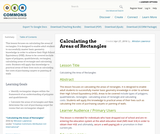Unrestricted Use
CC BY
Rating
0.0 stars

This lesson focuses on calculating the areas of rectangles. It is designed to enable adult students to successfully master basic geometry knowledge in order to achieve their High School Equivalency (HSE). Areas to be covered include types of polygons, quadrilaterals, rectangles; calculating areas of rectangle and calculating costs. Students will apply this knowledge to practical areas of their lives such as calculating the costs of purchasing carpets or painting of walls

Subject:
Mathematics
Material Type:
Interactive
Lesson Plan
Author:
Winston Lawrence
Date Added:
05/05/2016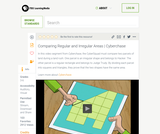Read the Fine Print
Educational Use
Rating
3.0 stars

The CyberSquad proves that the area of Hacker's land is equal to the area of Judge Trudy's land in this video segment from Cyberchase.

Subject:
Mathematics
Material Type:
Lecture
Provider:
PBS LearningMedia
Provider Set:
PBS Learning Media: Multimedia Resources for the Classroom and Professional Development
Author:
U.S. Department of Education
WNET
Date Added:
07/08/2008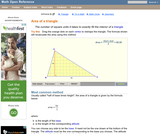Read the Fine Print
Rating
0.0 stars

An interactive applet and associated web page that explain the area of a triangle. The applet shows a triangle that can be reshaped by dragging any vertex. As it changes, the area is continually recalculated using the 'half base times height' method. The triangle has a fixed square grid in its interior that can be used to visually estimate the area for later correlation with the calculated value. The calculation can be hidden while estimation is in progress. The text page has links to a similar page that uses Heron's Formula to compute the area. Applet can be enlarged to full screen size for use with a classroom projector. This resource is a component of the Math Open Reference Interactive Geometry textbook project at http://www.mathopenref.com.

Subject:
Geometry
Material Type:
Reading
Simulation
Provider:
Math Open Reference
Author:
John Page
Date Added:
02/16/2011Only Sharing Permitted
CC BY-NC-ND
Rating
4.5 stars

This lesson unit is intended to help teachers assess how well students are able to visualize two-dimensional cross-sections of representations of three-dimensional objects. In particular, the lesson will help you identify and help students who have difficulties recognizing and drawing two-dimensional cross-sections at different points along a plane of a representation of a three-dimensional object.

Subject:
Geometry
Material Type:
Assessment
Lesson Plan
Provider:
Shell Center for Mathematical Education
Provider Set:
Mathematics Assessment Project (MAP)
Date Added:
04/26/2013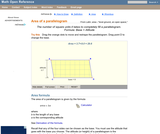Read the Fine Print
Rating
0.0 stars

A web page and interactive applet showing the ways to calculate the area of a parallelogram. The user can drag the vertices of the parallelogram and the other points change automatically to ensure it remains a parallelogram. A grid inside the shape allows students to estimate the area visually, then check against the actual computed area, which is continuously recomputed and displayed. The text on the page gives three different ways to calculate the area with a formula for each. The applet uses one of the methods to compute the area in real time, so it changes as the rhombus is reshaped with the mouse. A companion page is http://www.mathopenref.com/parallelogram.html showing the definition and properties of a parallelogram Applet can be enlarged to full screen size for use with a classroom projector. This resource is a component of the Math Open Reference Interactive Geometry textbook project at http://www.mathopenref.com.

Subject:
Geometry
Material Type:
Reading
Simulation
Provider:
Math Open Reference
Author:
John Page
Date Added:
02/16/2011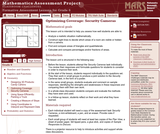Only Sharing Permitted
CC BY-NC-ND
Rating
0.0 stars

This lesson unit is intended to help sixth grade teachers assess how well students are able to: Analyze a realistic situation mathematically; construct sight lines to decide which areas of a room are visible or hidden from a camera; find and compare areas of triangles and quadrilaterals; and calculate and compare percentages and/or fractions of areas.

Subject:
Geometry
Ratios and Proportions
Material Type:
Assessment
Lesson Plan
Provider:
Shell Center for Mathematical Education
Provider Set:
Mathematics Assessment Project (MAP)
Date Added:
04/26/2013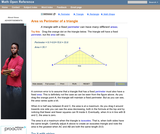Read the Fine Print
Rating
4.0 stars

An interactive applet and associated web page that show the relationship between the perimeter and area of a triangle. It shows that a triangle with a constant perimeter does NOT have a constant area. The applet has a triangle with one vertex draggable and a constant perimeter. As you drag the vertex, it is clear that the area varies, even though the perimeter is constant. Optionally, you can see the path traced by the dragged vertex and see that it forms an ellipse. A link takes you to a page where this effect is exploited to construct an ellipse with string and pins. The applet can be enlarged to full screen size for use with a classroom projector. This resource is a component of the Math Open Reference Interactive Geometry textbook project at http://www.mathopenref.com.

Subject:
Geometry
Material Type:
Reading
Simulation
Provider:
Math Open Reference
Author:
John Page
Date Added:
02/16/2011Rating
0.0 stars

Surface Area and Volume

Type of Unit: Conceptual

Prior Knowledge

Students should be able to:

Identify rectangles, parallelograms, trapezoids, and triangles and their bases and heights.
Identify cubes, rectangular prisms, and pyramids and their faces, edges, and vertices.
Understand that area of a 2-D figure is a measure of the figure's surface and that it is measured in square units.
Understand volume of a 3-D figure is a measure of the space the figure occupies and is measured in cubic units.

Lesson Flow

The unit begins with an exploratory lesson about the volumes of containers. Then in Lessons 2–5, students investigate areas of 2-D figures. To find the area of a parallelogram, students consider how it can be rearranged to form a rectangle. To find the area of a trapezoid, students think about how two copies of the trapezoid can be put together to form a parallelogram. To find the area of a triangle, students consider how two copies of the triangle can be put together to form a parallelogram. By sketching and analyzing several parallelograms, trapezoids, and triangles, students develop area formulas for these figures. Students then find areas of composite figures by decomposing them into familiar figures. In the last lesson on area, students estimate the area of an irregular figure by overlaying it with a grid. In Lesson 6, the focus shifts to 3-D figures. Students build rectangular prisms from unit cubes and develop a formula for finding the volume of any rectangular prism. In Lesson 7, students analyze and create nets for prisms. In Lesson 8, students compare a cube to a square pyramid with the same base and height as the cube. They consider the number of faces, edges, and vertices, as well as the surface area and volume. In Lesson 9, students use their knowledge of volume, area, and linear measurements to solve a packing problem.

Subject:
Mathematics
Geometry
Material Type:
Unit of Study
Provider:
Pearson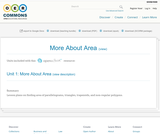Unrestricted Use
CC BY
Rating
0.0 stars

Lesson plans on finding area of parallelograms, triangles, trapezoids, and non-regular polygons.

Subject:
Mathematics
Material Type:
Homework/Assignment
Lesson Plan
Author:
Amy Whitchurch
Date Added:
01/28/2016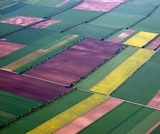Conditional Remix & Share Permitted
CC BY-NC
Rating
0.0 stars

Lesson OverviewStudents use what they know about finding the areas of basic figures to find areas of composite figures.Key ConceptsA composite figure is a figure that can be divided into two or more basic figures.The area of a composite figure can be found by dividing it into basic figures whose areas can be calculated easily.For some figures, the area can also be found by surrounding the figure with a basic figure, creating other basic figures “between” the original figure and the surrounding figure. The area of the original figure can then be found by subtracting the basic figure.Goals and Learning ObjectivesFind the area of composite figures by decomposing and composing them into more basic figures.

Subject:
Geometry
Material Type:
Lesson Plan
Provider:
Pearson
Date Added:
09/21/2015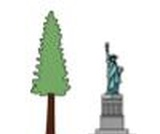Conditional Remix & Share Permitted
CC BY-NC
Rating
0.0 stars

This course includes links to Grade 6 Unit 3 of GeoGebra's interactive apps that allows teachers to virtually look over the shoulder of their students as they’re working Illustrative Mathematics problems in their video conferencing classroom. Moodle adds asynchronous communications between students and teachers - and between students, and several ways to assess student learning.

On the MoodleNet site click on the title of the course which will then be downloaded to your computer. The courses can be uploaded into Schoology, Canvas, Moodle, and D2L.

Subject:
Mathematics
Material Type:
Full Course
Author:
Dan McGuire
Diana Fisher Ph.D.
Date Added:
04/07/2021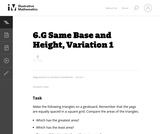Unrestricted Use
CC BY
Rating
0.0 stars

This is the first version of a task asking students to find the areas of triangles that have the same base and height, and is the most concrete.

Subject:
Mathematics
Geometry
Material Type:
Activity/Lab
Provider:
Illustrative Mathematics
Provider Set:
Illustrative Mathematics
Author:
Illustrative Mathematics
Date Added:
05/01/2012Only Sharing Permitted
CC BY-NC-ND
Rating
4.0 stars

This lesson unit is intended to help you assess how well students are able to: Perform arithmetic operations, including those involving whole-number exponents, recognizing and applying the conventional order of operations; Write and evaluate numerical expressions from diagrammatic representations and be able to identify equivalent expressions; apply the distributive and commutative properties appropriately; and use the method for finding areas of compound rectangles.

Subject:
Geometry
Material Type:
Assessment
Lesson Plan
Provider:
Shell Center for Mathematical Education
Provider Set:
Mathematics Assessment Project (MAP)
Date Added:
04/26/2013Conditional Remix & Share Permitted
CC BY-NC
Rating
0.0 stars

Subject:
Mathematics
Material Type:
Full Course
Provider:
Pearson
Date Added:
10/06/2016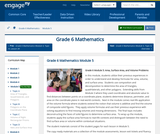Conditional Remix & Share Permitted
CC BY-NC-SA
Rating
0.0 stars

In this module, students utilize their previous experiences in order to understand and develop formulas for area, volume, and surface area.  Students use composition and decomposition to determine the area of triangles, quadrilaterals, and other polygons.  Extending skills from Module 3 where they used coordinates and absolute value to find distances between points on a coordinate plane, students determine distance, perimeter, and area on the coordinate plane in real-world contexts.  Next in the module comes real-life application of the volume formula where students extend the notion that volume is additive and find the volume of composite solid figures.  They apply volume formulas and use their previous experience with solving equations to find missing volumes and missing dimensions.  The final topic includes deconstructing the faces of solid figures to determine surface area.  To wrap up the module, students apply the surface area formula to real-life contexts and distinguish between the need to find surface area or volume within contextual situations.

Subject:
Geometry
Material Type:
Module
Provider:
New York State Education Department
Provider Set:
EngageNY
Date Added:
01/31/2014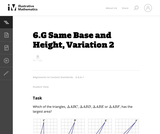Unrestricted Use
CC BY
Rating
0.0 stars

This is the second version of a task asking students to find the areas of triangles that have the same base and height. This presentation is more abstract as students are not using physical models.

Subject:
Mathematics
Geometry
Material Type:
Activity/Lab
Provider:
Illustrative Mathematics
Provider Set:
Illustrative Mathematics
Author:
Illustrative Mathematics
Date Added:
05/01/2012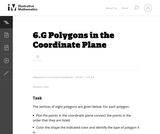Unrestricted Use
CC BY
Rating
0.0 stars

This is a task from the Illustrative Mathematics website that is one part of a complete illustration of the standard to which it is aligned. Each task has at least one solution and some commentary that addresses important asects of the task and its potential use. Here are the first few lines of the commentary for this task: The vertices of eight polygons are given below. For each polygon: * Plot the points in the coordinate plane connect the points in the order that they a...

Subject:
Mathematics
Material Type:
Activity/Lab
Provider:
Illustrative Mathematics
Provider Set:
Illustrative Mathematics
Author:
Illustrative Mathematics
Date Added:
09/08/2013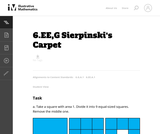Unrestricted Use
CC BY
Rating
0.0 stars

This is a task from the Illustrative Mathematics website that is one part of a complete illustration of the standard to which it is aligned. Each task has at least one solution and some commentary that addresses important asects of the task and its potential use. Here are the first few lines of the commentary for this task: Take a square with area 1. Divide it into 9 equal-sized squares. Remove the middle one. What is the area of the figure now? Take the remaining 8 square...

Subject:
Mathematics
Material Type:
Activity/Lab
Provider:
Illustrative Mathematics
Provider Set:
Illustrative Mathematics
Author:
Illustrative Mathematics
Date Added:
08/06/2013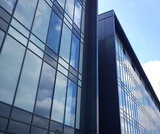Conditional Remix & Share Permitted
CC BY-NC
Rating
0.0 stars

Lesson OverviewStudents find the area of a parallelogram by rearranging it to form a rectangle. They find the area of a trapezoid by putting together two copies of it to form a parallelogram. By doing these activities and by analyzing the dimensions and areas of several examples of each figure, students develop and understand area formulas for parallelograms and trapezoids.Key ConceptsA parallelogram is a quadrilateral with two pairs of parallel sides. The base of a parallelogram can be any of the four sides. The height is the perpendicular distance from the base to the opposite side.A trapezoid is a quadrilateral with exactly one pair of parallel sides. The bases of a trapezoid are the parallel sides. The height is the perpendicular distance between the bases.You can cut a parallelogram into two pieces and reassemble them to form a rectangle. Because the area does not change, the area of the rectangle is the same as the area of the parallelogram. This gives the parallelogram area formula A = bh.You can put two identical trapezoids together to form a parallelogram with the same height as the trapezoid and a base length equal to the sum of the base lengths of the trapezoid. The area of the parallelogram is (b1 + b2)h, so the area of the trapezoid is one-half of this area. Thus, the trapezoid area formula is A = 12(b1 + b2)h.Goals and Learning ObjectivesDevelop and explore the formula for the area of a parallelogram.Develop and explore the formula for the area of a trapezoid.

Subject:
Geometry
Material Type:
Lesson Plan
Provider:
Pearson
Date Added:
09/21/2015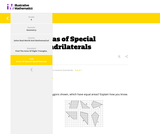Conditional Remix & Share Permitted
CC BY-NC-SA
Rating
0.0 stars

This task asks students to identify which of the six polygons have the same area. Students may complete the task using a variety of techniques including decomposing shapes, using transformations (rotations, reflections, translations) to move one or more parts of the figure to another part to more easily calculate the area, enclosing the polygon inside a larger rectangle and then subtract the areas of the "extra" pieces, etc.

Subject:
Mathematics
Material Type:
Activity/Lab
Diagram/Illustration
Lesson Plan
Provider:
Illustrative Mathematics
Date Added:
05/08/2020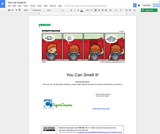Conditional Remix & Share Permitted
CC BY-NC
Rating
0.0 stars

Students will have to solve the real world problem of locker smell leakage by building an air filter that will cover the vents on the top of a locker. This project goes well with a curriculum on the particle nature of gases and phase changes.

Subject:
Engineering
Measurement and Data
Chemistry
Material Type:
Activity/Lab
Lesson Plan
Provider:
Lane County STEM Hub
Provider Set:
Content in Context SuperLessons
Author:
Allison Machado
Chris Michael
Date Added:
06/27/2017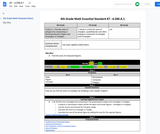Conditional Remix & Share Permitted
CC BY-NC-SA
Rating
0.0 stars

Find the area of polygons by composing or decomposing the shapes into rectangles or triangles.

Subject:
Mathematics
Material Type:
Activity/Lab
Author:
Liberty Public Schools
Date Added:
04/12/2021
Remix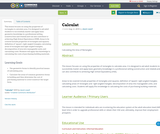Unrestricted Use
CC BY
Rating
0.0 stars

This lesson focuses on using the properties of rectangles to calculate area. It is designed to aid adult students to successfully master and apply basic geometry knowledge in a professional setting (construction and related) and can also contribute to achieving High School Equivalency (HSE).
Areas to be covered include properties of rectangles and squares; definition of ‘square’; right-angled triangles; calculating areas of rectangles and right-angled triangles; decomposition of area into manageable units; and calculating costs. Students will apply this knowledge to calculating the costs of purchasing building materials.

Subject:
Mathematics
Material Type:
Interactive
Lesson Plan
Author:
david cowell
Date Added:
08/08/2019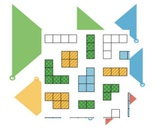Conditional Remix & Share Permitted
CC BY-NC
Rating
0.0 stars

This course includes links to Grade 6 Unit 2 of GeoGebra's interactive apps that allows teachers to virtually look over the shoulder of their students as they’re working Illustrative Mathematics problems in their video conferencing classroom. Moodle adds asynchronous communications between students and teachers - and between students, and several ways to assess student learning.

On the MoodleNet site click on the title of the course which will then be downloaded to your computer. The courses can be uploaded into Schoology, Canvas, Moodle, and D2L

Subject:
Mathematics
Material Type:
Full Course
Author:
Dan MGuire
Diana Fisher Ph.D.
Date Added:
04/07/2021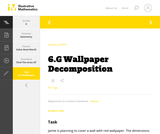Unrestricted Use
CC BY
Rating
0.0 stars

This is a task from the Illustrative Mathematics website that is one part of a complete illustration of the standard to which it is aligned. Each task has at least one solution and some commentary that addresses important aspects of the task and its potential use.

Subject:
Geometry
Material Type:
Activity/Lab
Provider:
Illustrative Mathematics
Provider Set:
Illustrative Mathematics
Author:
Illustrative Mathematics
Date Added:
08/06/2015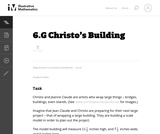Unrestricted Use
CC BY
Rating
0.0 stars

This task is primarily about volume and surface area, although it also gives students an early look at converting between measurements in scale models and the real objects they correspond to.

Subject:
Mathematics
Geometry
Material Type:
Activity/Lab
Provider:
Illustrative Mathematics
Provider Set:
Illustrative Mathematics
Author:
Illustrative Mathematics
Date Added:
05/01/2012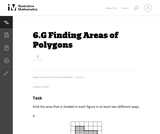Unrestricted Use
CC BY
Rating
0.0 stars

The purpose of this task is to have students work on a sequence of area problems that shows the advantage of increasingly abstract strategies in preparation for developing general area formulas for parallelograms and triangles.

Subject:
Mathematics
Geometry
Material Type:
Activity/Lab
Provider:
Illustrative Mathematics
Provider Set:
Illustrative Mathematics
Author:
Illustrative Mathematics
Date Added:
05/01/2012Only Sharing Permitted
CC BY-NC-ND
Rating
4.0 stars

This lesson unit is intended to help teachers assess how well students are able to: Select appropriate mathematical methods to use for an unstructured problem; interpret a problem situation, identifying constraints and variables, and specify assumptions; work with 2- and 3-dimensional shapes to solve a problem involving capacity and surface area; and communicate their reasoning clearly.

Subject:
Geometry
Material Type:
Assessment
Lesson Plan
Provider:
Shell Center for Mathematical Education
Provider Set:
Mathematics Assessment Project (MAP)
Date Added:
04/26/2013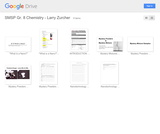Conditional Remix & Share Permitted
CC BY-NC
Rating
0.0 stars

In this unit of study, students will research topics in nanotechnology and attempt to identify a mystery mixture of different powders using a scanning-electron microscope. This unit integrates nine STEM attributes and was developed as part of the South Metro-Salem STEM Partnership's Teacher Leadership Team. Any instructional materials are included within this unit of study.

Subject:
Physical Science
Material Type:
Activity/Lab
Assessment
Homework/Assignment
Lecture Notes
Lesson Plan
Reading
Unit of Study
Provider:
South Metro-Salem STEM Partnership
Author:
Larry Zurcher
Date Added:
04/29/2015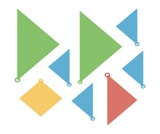Conditional Remix & Share Permitted
CC BY-NC
Rating
0.0 stars

This course includes links to Grade 6 Unit 1 of GeoGebra's interactive apps that allows teachers to virtually look over the shoulder of their students as they’re working Illustrative Mathematics problems in their video conferencing classroom. Moodle adds asynchronous communications between students and teachers - and between students, and several ways to assess student learning.

From the MoodleNet site click on the title of the course which will then be downloaded to your computer. The courses can be uploaded into Schoology, Canvas, Moodle, and D2L.

Subject:
Mathematics
Material Type:
Full Course
Author:
Dan McGuire
Diana Fisher Ph.D.
Date Added:
04/07/2021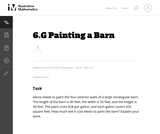Unrestricted Use
CC BY
Rating
0.0 stars

The purpose of this task is to provide students an opportunity to use mathematics addressed in different standards in the same problem.

Subject:
Mathematics
Geometry
Material Type:
Activity/Lab
Provider:
Illustrative Mathematics
Provider Set:
Illustrative Mathematics
Author:
Illustrative Mathematics
Date Added:
05/01/2012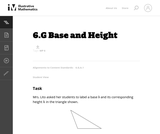Unrestricted Use
CC BY
Rating
0.0 stars

The purpose of this task is to help students understand what is meant by a base and its corresponding height in a triangle and to be able to correctly identify all three base-height pairs.

Subject:
Mathematics
Geometry
Material Type:
Activity/Lab
Provider:
Illustrative Mathematics
Provider Set:
Illustrative Mathematics
Author:
Illustrative Mathematics
Date Added:
05/01/2012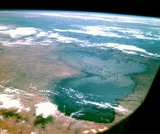Conditional Remix & Share Permitted
CC BY-NC
Rating
0.0 stars

Lesson OverviewStudents estimate the area of Lake Chad by overlaying a grid on the map of the lake.Key ConceptThe area of an irregular figure can be found by overlaying a grid on the figure. By estimating the number of grid squares the figure covers and multiplying by the area of each square, you can find the approximate area of the figure. The accuracy of the estimate depends on the size of the grid squares. Using a smaller grid leads to a more accurate estimate because more whole grid squares are completely filled. However, using a smaller grid also requires more counting and more combining of partially-filled squares and is, therefore, more time-consuming. Using a larger grid gives a quicker, but rougher, estimate of the area.Goals and Learning ObjectivesUse a grid to find the area of an irregular figure.MaterialsMap of Lake Chad handout (one for each pair of students)Rulers, optional (one for each pair of students)

Subject:
Geometry
Material Type:
Lesson Plan
Provider:
Pearson
Date Added:
09/21/2015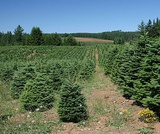Conditional Remix & Share Permitted
CC BY-NC
Rating
0.0 stars

Lesson OverviewStudents find the area of a triangle by putting together a triangle and a copy of the triangle to form a parallelogram with the same base and height as the triangle. Students also create several examples of triangles and look for relationships among the base, height, and area measures. These activities lead students to develop and understand a formula for the area of a triangle.Key ConceptsTo find the area of a triangle, you must know the length of a base and the corresponding height. The base of a triangle can be any of the three sides. The height is the perpendicular distance from the vertex opposite the base to the line containing the base. The height can be found inside or outside the triangle, or it can be the length of one of the sides.You can put together a triangle and a copy of the triangle to form a parallelogram with the same base and height as the triangle. The area of the original triangle is half of the area of the parallelogram. Because the area formula for a parallelogram is A = bh, the area formula for a triangle is A = 12bh.Goals and Learning ObjectivesDevelop and explore the formula for the area of a triangle.

Subject:
Geometry
Material Type:
Lesson Plan
Provider:
Pearson
Date Added:
09/21/2015